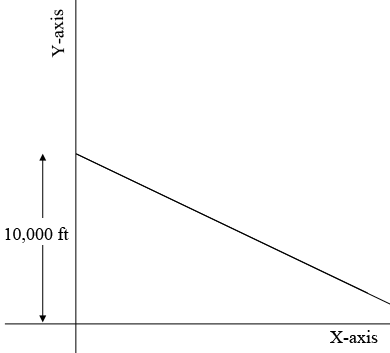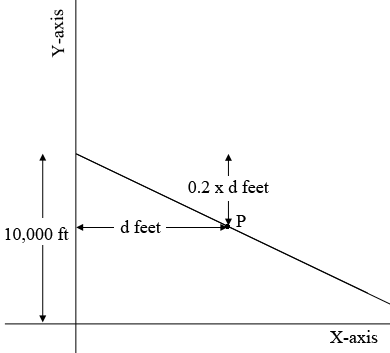SEARCH HOMEMath Central Quandaries & QueriesQuestion from Hannah, a student: The information provided is ---A ski slope has a slope of -0.2. You start at an altitude of 10,000ft. Then the question is "Write the equation giving altitude (a) as a function of horizontal distance moved (d). I know this is probably a very simple question but I didn't take a math class my senior year and now I'm a freshman in college, so some of it is very frustrating to me. Any help is much appreciated, thank you!Hi Hannah,

I am going to put a coordinate system on the plane with the X-axis at an altitude of 0 feet and the origin directly below the peak of the hill. The fact that the slope is negative means that the slope is downwards to the right and so here is my diagram.The fact that the slope is $-0.2$ means that if you are on the slope and move 1 unit in the X direction then your altitude decreases by $0.2$ units. Your units are feet and hence if you move $d$ feet in the X direction your altitude changes by $-0.2 \times d$ feet.Thus if you are on the slope at the point $P$ which is $d$ feet to the right of the Y-axis then you are $0.2 \times d$ feet below the 10,000 foot point. Thus the coordinates of $P$ are $d$ and $10,000 - 0.2d$ feet.

I hope this helps,
PennyMath Central is supported by the University of Regina and the Imperial Oil Foundation.